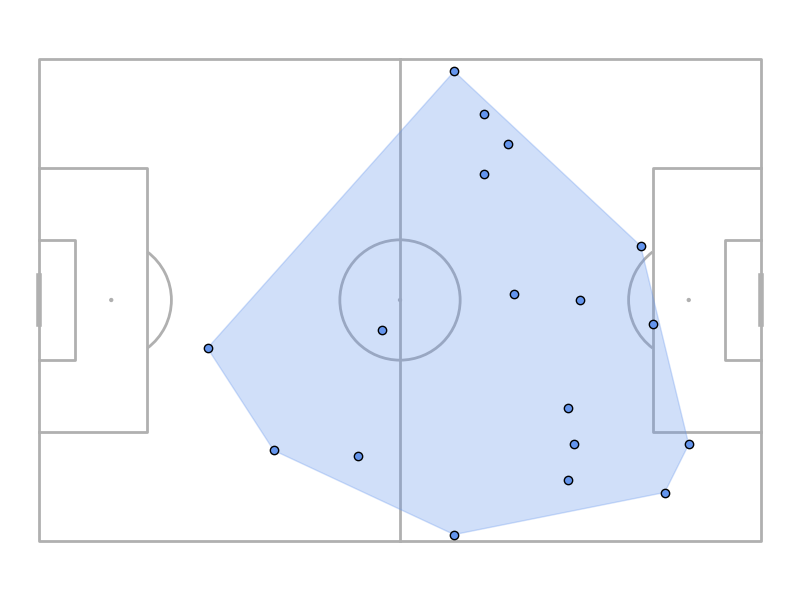# Convex Hull

This example shows how to plot a convex hull around a player’s events.

Thanks to Devin Pleuler for adding this to mplsoccer.

```from mplsoccer import Pitch, Sbopen
import matplotlib.pyplot as plt

parser = Sbopen()
df, related, freeze, tactics = parser.event(7478)
```

Filter passes by Jodie Taylor

```df = df[(df.player_name == 'Jodie Taylor') & (df.type_name == 'Pass')].copy()
```

Plotting

```pitch = Pitch()
fig, ax = pitch.draw(figsize=(8, 6))
hull = pitch.convexhull(df.x, df.y)
poly = pitch.polygon(hull, ax=ax, edgecolor='cornflowerblue', facecolor='cornflowerblue', alpha=0.3)
scatter = pitch.scatter(df.x, df.y, ax=ax, edgecolor='black', facecolor='cornflowerblue')
plt.show()  # if you are not using a Jupyter notebook this is necessary to show the plot
```Total running time of the script: ( 0 minutes 0.938 seconds)

Gallery generated by Sphinx-Gallery